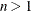### More on Index Sets

Dummy parameters behave like parameters but are assigned values only when an index set is evaluated. You can reference the declared dummy parameters from index set expressions that follow the index set item. You can also reference the dummy parameters in the expression or statement controlled by the index set. As the members of the set expression of an index set item are enumerated, the element values of the members are assigned to the local dummy parameters.

The number of names in a dummy parameter declaration must match the element length of the corresponding set expression in the index set item. A single name is allowed when the set member type is scalar (numeric or string). If the set members are tuples that haveelements, thennames are required between the angle brackets (< >) that precede the IN keyword.

Multiple index set items in an index set are nominally processed in a left-to-right order. That is, a set expression from an index set item is evaluated as though the index set items that precede it have already been evaluated. The left-hand index set items can assign values to local dummy parameters that are used by the set expressions that follow them. After each member from the set expression is enumerated, any index set items to the right are reevaluated as needed. The actual order in which index set items are evaluated can vary, if necessary, to allow more efficient enumeration. PROC OPTMODEL generates the same set of values in any case, although possibly in a different order than strict left-to-right evaluation.

You can view the element combinations that are generated from an index set as tuples. This is especially true for index set expressions (see the section Index Set Expression). However, in most cases no tuple set is actually formed, and the element values are assigned only to local dummy parameters.

You can specify a selection expression following a colon (:). The index set generates only those combinations of values for which the selection expression is true. For example, the following statements produce a set of upper triangular indices:

```proc optmodel;
put (setof {i in 1..3, j in 1..3 : j >= i} <i, j>);
```

These statements produce the output in Figure 5.63.

Figure 5.63: Upper Triangular Index Set

 ```{<1,1>,<1,2>,<1,3>,<2,2>,<2,3>,<3,3>} ```

You can use the left-to-right evaluation of index set items to express the previous set more compactly. The following statements produce the same output as the previous statements:

```proc optmodel;
put ({i in 1..3, i..3});
```

In this example, the first time the second index set item is evaluated, the value of the dummy parameter `i` is 1, so the item produces the set {1,2,3}. At the second evaluation the value of `i` is 2, so the second item produces the set {2,3}. At the final evaluation the value of `i` is 3, so the second item produces the set {3}.

In many cases it is useful to combine the SLICE operator with index sets. A special form of index set item uses the SLICE operator implicitly. Normally an index set item that is applied to a set of tuples of length greater than one must be of the form

< name-1,name-n> IN set-expression

In the special form, one or more of the name elements are replaced by expressions. The expressions select tuple elements by using the SLICE operator. An expression that consists of a single name must be enclosed in parentheses to distinguish it from a dummy parameter. The remaining names are the dummy parameters for the index set item that is applied to the SLICE result. The following example demonstrates the use of implicit set slicing:

```proc optmodel;
number N = 3;
set<num,str> S = {<1,'a'>,<2,'b'>,<3,'a'>,<4,'b'>};
put ({i in 1..N, <(i),j> in S});
put ({i in 1..N, j in slice(<i,*>, S)});
```

The two PUT statements in this example are equivalent.